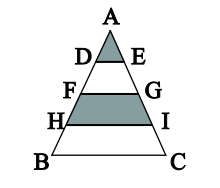###### back to index | new

What is the radius of a circle inscribed in a triangle with sides of length $5$, $12$ and $13$ units?

How many collections of six positive, odd integers have a sum of $18$? Note that $1 + 1 + 1 + 3 + 3 + 9$ and $9 + 1 + 3 + 1 + 3 + 1$ are considered to be the same collection.

In $\triangle{ABC}$, segments AB and AC have each been divided into four congruent segments. We must find the fraction of the triangle that is shaded.